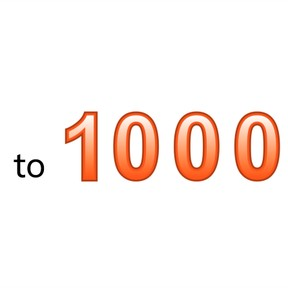Know numbers to 1,000

# Know numbers to 1,000

Know numbers to 1,000

No account needed.8,000 schools use Gynzy92,000 teachers use Gynzy1,600,000 students use Gynzy

## General

Students learn to recognize numbers 21 to 100 from their spoken form and can write down the numbers. Students can say the numbers, write the numbers, and write the numbers in words.

2.NBT.A.3

## Relevance

Discuss with students that numbers are all around us and it is important to recognize them. An example is if you go on vacation and need to find the number of your hotel room.

## Introduction

Students practice writing numbers to 100. They write down the number from the number in words. Say the numbers aloud. Check the answers on the interactive whiteboard.

## Development

Point at the hundreds to 1,000 and say them aloud. Ask students to repeat the numbers after you. Next practice saying a selection of numbers to 1,000 aloud. Check that students understand the link between the number written in words and the number. Explain how to write the number using 635 and 653 as examples. They can sometimes be mixed up. Point out that in writing, you first write the number in the hundreds place, then the tens place and finally the ones place. Have students practice this by writing a selection of numbers. Examples are 423, 760, 133, 878, 554. Write the correct answer on the interactive whiteboard.

Check that students have understood by asking the following questions:
Which number do you write first for 723?
Which number do you write last for 548?
What order do you write the numbers in for numbers to 1,000?

## Guided Practice

Students practice by first linking the visual number to the spoken number. Next by linking a spoken number to a written, and finally by linking a spoken number to a visual number.

## Closing

Discuss why it is useful to know numbers to 1,000 with the students. Numbers are all around us. An example is if you are on vacation and need to find your hotel room. Check that students understood by having them say the numbers on the interactive whiteboard out loud.

## Teaching Tip

Students who have difficulty can be asked to practice speaking and writing numbers to 100. Students who need some challenge can be encouraged to try saying and writing numbers to 10,000.

### The online teaching platform for interactive whiteboards and displays in schools

• Save time building lessons

• Manage the classroom more efficiently

• Increase student engagement